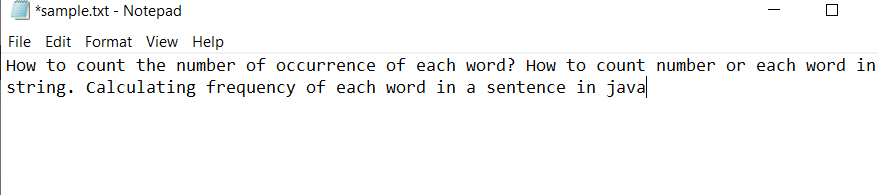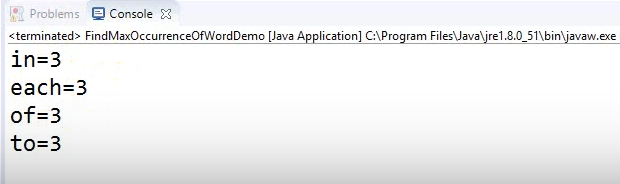Open in App
Not now

# Java Program to Find the Most Repeated Word in a Text File

• Last Updated : 20 Feb, 2023

Map and Map.Entry interface will be used as the Map interface maps unique keys to values. A key is an object that is used to retrieve a value at a later date. The Map.Entry interface enables you to work with a map entry. Also, we will use the HashMap class to store items in “key/value” pairs and access them by an index of another type.

Illustration:

Suppose the content of the sample text file is as follows:

Input: A text file containing sequence of arbitrary words

“How to count the number of occurrence of each word? How to count number or each word in string.  Calculating frequency of each word in a sentence in java.”

Output: List of words that have the maximum occurrence

```in = 3
each = 3
of = 3
to = 3```

Implementation: Sample file input image is as follows:Example

## Java

 `// Java Program to Find the` `// Most Repeated Word in a Text File`   `// Importing File classes` `import` `java.io.File;` `import` `java.io.FileNotFoundException;` `// Importing Map and HashMap class from` `// java.util package` `import` `java.util.HashMap;` `import` `java.util.Map;` `import` `java.util.Map.Entry;` `// Importing Scanner class to` `// take input from the user` `import` `java.util.Scanner;`   `// Class ` `// To find maximum occurrences` `public` `class` `GFG {`   `    ``// Method 1 - getWords()` `    ``// Reading out words from the file and` `    ``// mapping key value pair corresponding to` `    ``// each different word` `    ``static` `void` `getWords(String fileName,` `                         ``Map words)` `        ``throws` `FileNotFoundException` `    ``{` `        ``// Creating a Scanner class object` `        ``Scanner file = ``new` `Scanner(``new` `File(fileName));`   `        ``// Condition check using hasNext() method which` `        ``// holds true till there is word being read from the` `        ``// file. ` `      ``// As the end of file content,condition violates` `        ``while` `(file.hasNext()) {`   `            ``// Reading word using next() method` `            ``String word = file.next();`   `            ``// Frequency count variable` `            ``Integer count = words.get(word);`   `            ``// If the same word is repeating` `            ``if` `(count != ``null``) {`   `                ``// Incrementing corresponding count by unity` `                ``// every time it repeats` `              ``// while reading from the file` `                ``count++;` `            ``}` `            ``else`   `                ``// If word never occurred after occurring` `                ``// once, set count as unity` `                ``count = ``1``;` `            ``words.put(word, count);` `        ``}`   `        ``// Close the file and free up the resources` `        ``file.close();` `    ``}`   `    ``// Method 2 - getMaxOccurrence()` `    ``// To get maximum occurred Word` `    ``static` `int` `getMaxOccurrence(Map words)` `    ``{` `        ``// Initially set maximum count as unity` `        ``int` `max = ``1``;`   `        ``// Iterating over above Map using for-each loop` `        ``for` `(Entry word :` `             ``words.entrySet()) {`   `            ``// Condition check` `            ``// Update current max value  with the value` `            ``// exceeding unity in Map while traversing` `            ``if` `(word.getValue() > max) {` `                ``max = word.getValue();` `            ``}` `        ``}`   `        ``// Return the maximum value from the Map` `        ``return` `max;` `    ``}`   `    ``// Method 3` `    ``// Main driver method` `    ``public` `static` `void` `main(String[] args)` `        ``throws` `FileNotFoundException` `    ``{` `        ``// Creating an object of type Map` `        ``// Declaring object of String and Integer types` `        ``Map words` `            ``= ``new` `HashMap();`   `        ``// Retrieving the path as parameter to Method1()` `        ``// above to get the file to be read` `        ``getWords(``"C:\\Users\\dell\\sample.txt"``, words);`   `        ``// Variable holding the maximum` `        ``// repeated word count in a file` `        ``int` `max = getMaxOccurrence(words);`   `        ``// Traversing using for each loop` `        ``// Creating a set out of same elements` `        ``// contained in a HashMap` `        ``for` `(Entry word :` `             ``words.entrySet()) {`   `            ``// Comparing values using geValue() method` `            ``if` `(word.getValue() == max) {`   `                ``// Print and display word-count pair` `                ``System.out.println(word);` `            ``}` `        ``}` `    ``}`

Output:Time Complexity: O(n)
Auxiliary Space: O(n)

My Personal Notes arrow_drop_up
Related Articles